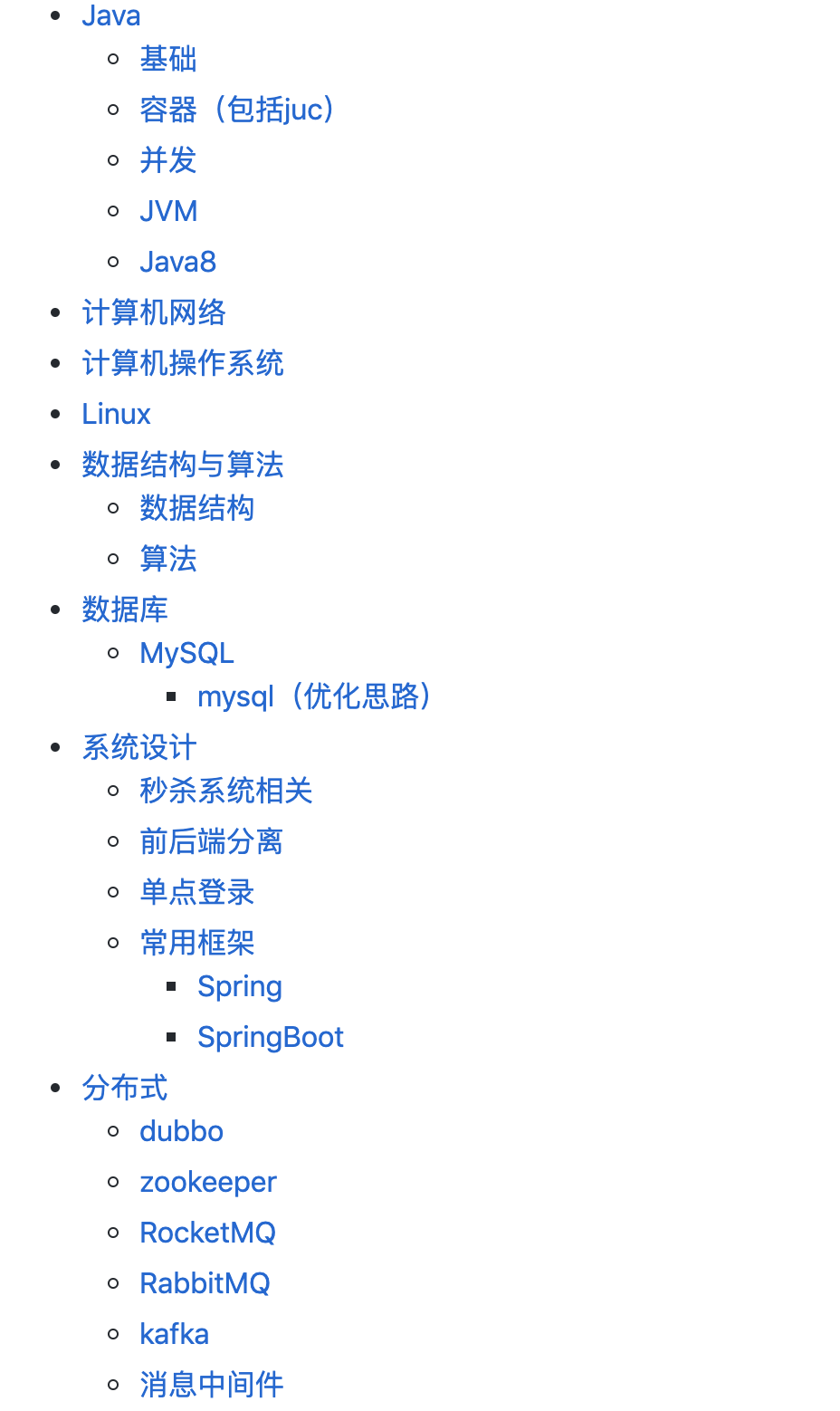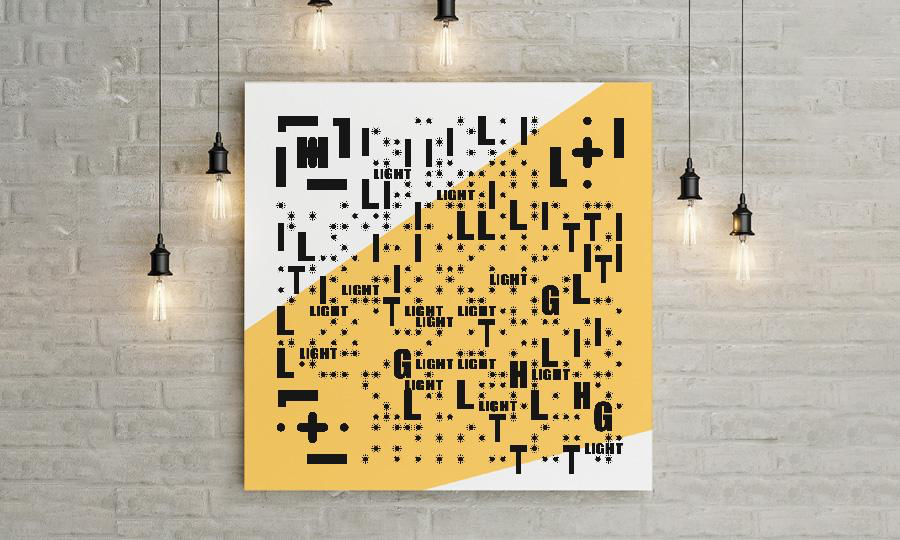# 蓝桥杯练习系统习题解答-入门训练

### 蓝桥杯练习系统习题-入门训练

> 入门训练 A+B问题

12 45

57

-10000 <= A, B <= 10000。

#include <stdio.h>

int main()
{
int A, B;

scanf("%d%d", &A, &B);
printf("%d", A+B);

return 0;
}

//参考代码Java
import java.io.IOException;

class Main
{
public static void main(String[] args) throws IOException
{
System.out.println(Integer.parseInt(args)+Integer.parseInt(args));
}
}


Fibonacci数列的递推公式为：Fn=Fn-1+Fn-2，其中F1=F2=1。

10

55

22

7704

1 <= n <= 1,000,000。

#include <stdio.h>
main()
{
unsigned long s=0,f1=1,f2=1,f3=1,n=0;
scanf("%d",&n);

if(n>2)
for(s=3;s<=n;s++)
{
f3=(f2+f1)%10007;
f1=f2;
f2=f3;
}

printf("%d",f3);
return 0;
}

//参考代码C语言
#include <stdlib.h>
#include <stdio.h>
#define MOD 10007
#define MAXN 1000001
int n, i, F[MAXN];
int main()
{
scanf("%d", &n);
F = 1;
F = 1;
for (i = 3; i <= n; ++i)
F[i] = (F[i-1] + F[i-2]) % MOD;
printf("%d\n", F[n]);
return 0;
}

//参考代码Java语言
import java.io.IOException;
public class Main {
public static void main(String[] args) throws IOException{
int n=Integer.valueOf(s);
int f1=1,f2=1,f3=0;
if(n<3){
System.out.print("1");
return;}
for(int i=3;i<=n;i++)
{if(f1>10007)f1=f1%10007;
if(f2>10007)f2=f2%10007;
f3=f1+f2;
f1=f2;
f2=f3;
}
System.out.print(f3%10007);
}

}



4

10

100

5050

1 <= n <= 1,000,000,000。

#include<stdio.h>
main()
{
long long n,s=0;

scanf("%I64d",&n);

s=(1+n)*n/2;

printf("%I64d",s);

return 0;
}

//参考代码C语言
#include <stdio.h>
int main()
{
int n;
long long ret = 0, tmp;
scanf("%d", &n);
tmp = n;
printf("%I64d", (1+tmp) * tmp / 2);
return 0;
}

//参考代码Java语言
import java.io.IOException;
public class Main {
public static void main(String args[]) throws NumberFormatException, IOException{
long sum = (1+i)*i/2;
System.out.println(sum);
}
}



4

50.2654825

1 <= r <= 10000。

#include <stdio.h>
#include <math.h>
#define PI atan(1.0)*4

main()
{
int r=0;
double s=0;
scanf("%d",&r);

s=PI*r*r;
printf("%.7f",s);

return 0;
}

//参考代码C语言
#include <stdio.h>
#include <math.h>

int main()
{
int r;
double s, PI;
scanf("%d", &r);
PI = atan(1.0) * 4;
s = PI * r * r;
printf("%.7lf", s);
return 0;
}

//参考代码Java语言
import java.io.IOException;
import java.math.BigDecimal;

70 71    public class Main {
public static void main(String[] args) throws IOException {
// TODO Auto-generated method stub
BigDecimal value = bg.multiply(bg.multiply(new BigDecimal(Math.PI))).setScale(7,BigDecimal.ROUND_HALF_UP);
System.out.println(value);
}
}#### 有收获？希望老铁们来个三连击，给更多的人看到这篇文章

1、老铁们，关注我的原创微信公众号「程序员的技术圈子」，专注于 Java、数据结构和算法、微服务、中间件等技术分享，保证你看完有所收获。

2、给俺点个赞呗，可以让更多的人看到这篇文章，顺便激励下我继续写作，嘻嘻。

3、另外，原创文章首发在我的个人博客：blog.ouyangsihai.cn，欢迎访问。

↓↓↓↓↓↓

04-25695401-03826
12-242301
09-02
03-185万+
02-042万+
03-235543
01-091265
01-162万+
11-20
03-25
01-262281
03-26
10-131万+
04-16705
©️2020 CSDN 皮肤主题: Age of Ai 设计师:meimeiellie点击重新获取扫码支付1.余额是钱包充值的虚拟货币，按照1:1的比例进行支付金额的抵扣。
2.余额无法直接购买下载，可以购买VIP、C币套餐、付费专栏及课程。余额充值